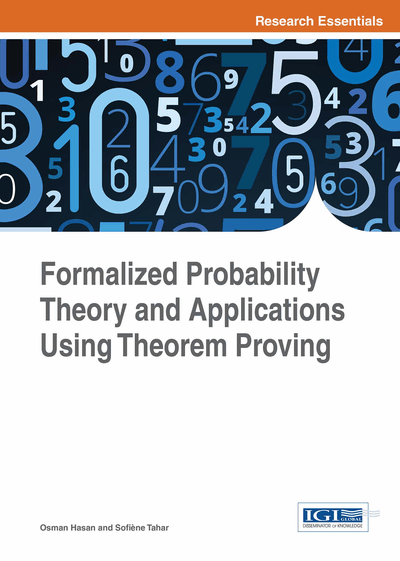# Discrete-Time Markov Chains in HOL

DOI: 10.4018/978-1-4666-8315-0.ch006
Available
\$37.50
No Current Special Offers

## Abstract

In this chapter, the authors describe the formalization of discrete-time Markov chains and the formal verification of some of its most important properties using the probability theory presented in the last chapter. In order to illustrate the usefulness of this work, a binary communication channel model and a collection process model involved in the Automatic Mail Quality Measurement (AMQM) protocol are formally analyzed in HOL.
Chapter Preview
Top

## 6.1 Formalization Of Discrete-Time Markov Chain

Given a probability space, a stochastic process { Xt: Ω → S }is defined as a sequence of random variables X, where t represents the time that can be discrete (represented by non-negative integers) or continuous (represented by real numbers). The set of values taken by each Xt, commonly called states, is referred to as the state space. The sample space of the process consists of all the possible state sequences based on a given state space S. Now, based on these definitions, a Markov process can be defined as a stochastic process with Markov property. If a Markov process has finite or countably infinite state space, then it is called a Markov chain and satisfies the following Markov property: for 0 ≤ t0≤ … ≤ tn and x0, …, xn+1in the state space then:

Pr { Xt+1 = xt+1 | Xt = xt,….., Xo = xo } = P { Xt+1 = xt+1 | Xt = xt } (6.1)

This mathematical equation expresses that the future state Xt+1 only depends on the current state Xt and is independent of the passed state Xi. This chapter mainly focuses on discrete-time Markov chains (DTMC) (Bhattacharya & Waymire (1990); Kannan, 1979)) with discrete state in a finite state space.

According to the Markov property, given in Equation (6.1) the future state is only dependent on the current state and is independent of all the other past states (Chung, 1960). The Markov property can be formalized as (Liu, 2013):

• Definition 6.1

```Ⱶ∀ X p s. mc_property X p s =
(∀t. random variable (X t) p s) ˄
∀ f t n. increasing_seq t˄P (∩k ∈ [ 0,n-1 ] { x | X tk x = f k }) ≠ 0(prob p ({ x | X tn+1 x = f (n + 1) } | { x | X tn x = f n }∩k ∈ [ 0,n-1 ] { x | X tk x = f k }) =
prob p ({ x | X tn+1 x = f (n + 1) } | { x | X tn x = f n }))```

where increasing_seq t defines the notion of increasing sequence, i.e.,∀ i j. i < j ⇒ t i < t j. The first conjunct indicates that the Markov property is based on a random process { Xt: Ω → S }. The quantified variable X represents a function of the random variables associated with time t which has the HOL4 type num or positive integer. This ensures the process is a discrete time random process. The random variables in this process are the functions built on the probability space p and a measurable space s. The conjunct P(∩k ∈ [ 0,n-1 ] { x | X tk x = f k }) ≠ 0 ensures that the corresponding conditional probabilities are well-defined, where f k returns the kth element of the state sequence.

We also have to explicitly mention all the usually implicit assumptions stating that the states belong to the considered space. The assumption P () ≠ 0 ensures that the corresponding conditional probabilities are well-defined, where f k returns the kth element of the state sequence.

In this chapter, P { x | X t x = i } represents prob p { x | (X t x = I) ˄ (x IN p_space p) }, where the term x IN p_space ensures that x is in the sample space of the considered probability space p. Similarly, the conditional probability P ({ x | X (t + 1) x = j } | { x | X t x = I }) represents cond_prob p ({ x | (X (t + 1) x = j) ˄ (x IN p_space p) } | { x | (X t x = I) ˄ (x IN p_space p) }) based on the formalization presented in the previous section.

## Complete Chapter List

Search this Book:
Reset